# Symmetry of Any Circle

Symmetry of Any Circle
Go back to  'Circles'

In this mini-lesson, we will learn about the symmetry of any circle by understanding the line of symmetry of any circle, and the type of symmetry in a circle, and how to apply them while solving problems. We will also discover interesting facts around them.

James and Filippa are sitting in the crowds and watching a thrilling game between Boston Celtics and New York Knicks.

James and Filippa are sitting in the middle of the crowd but on different sides, as shown in the picture below.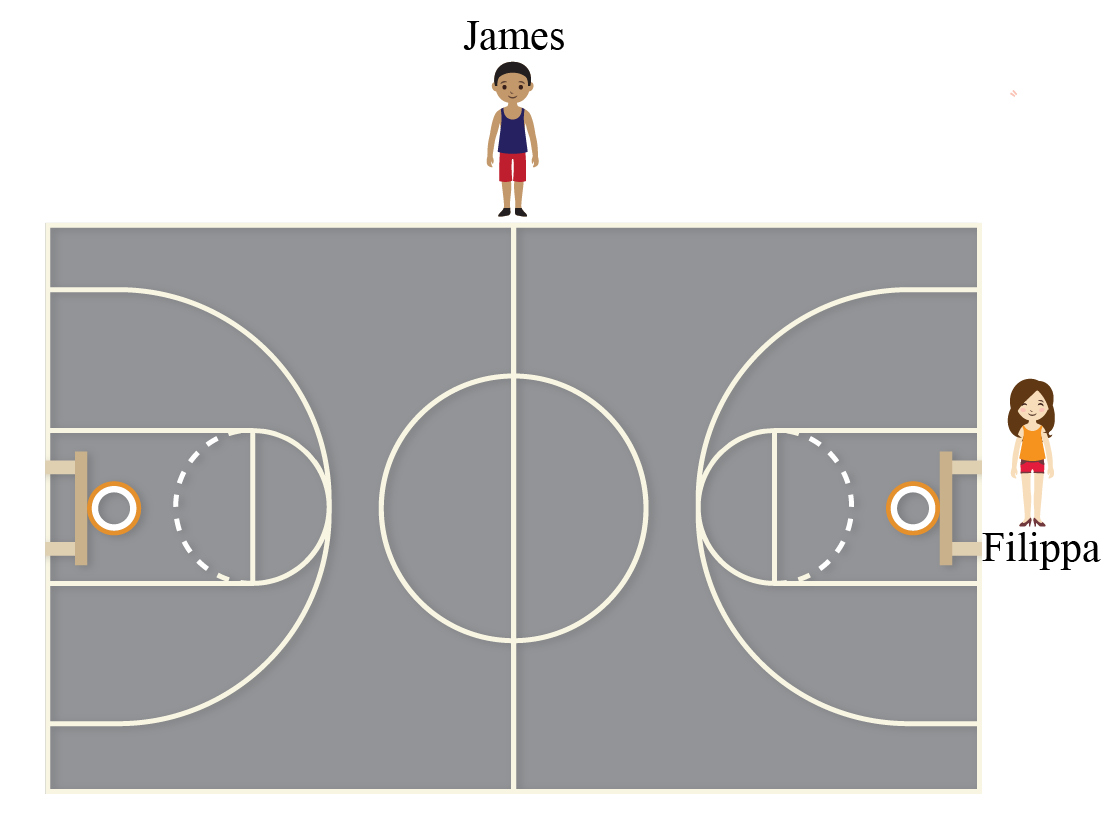After the match is over, James observed that if a vertical line is drawn from the middle of the court, it divides the court into two identical parts.

It means that the two parts of the court are symmetrical.

Filippa also observed that if a horizontal line is drawn from the middle of the court, it divides the court into two symmetrical parts.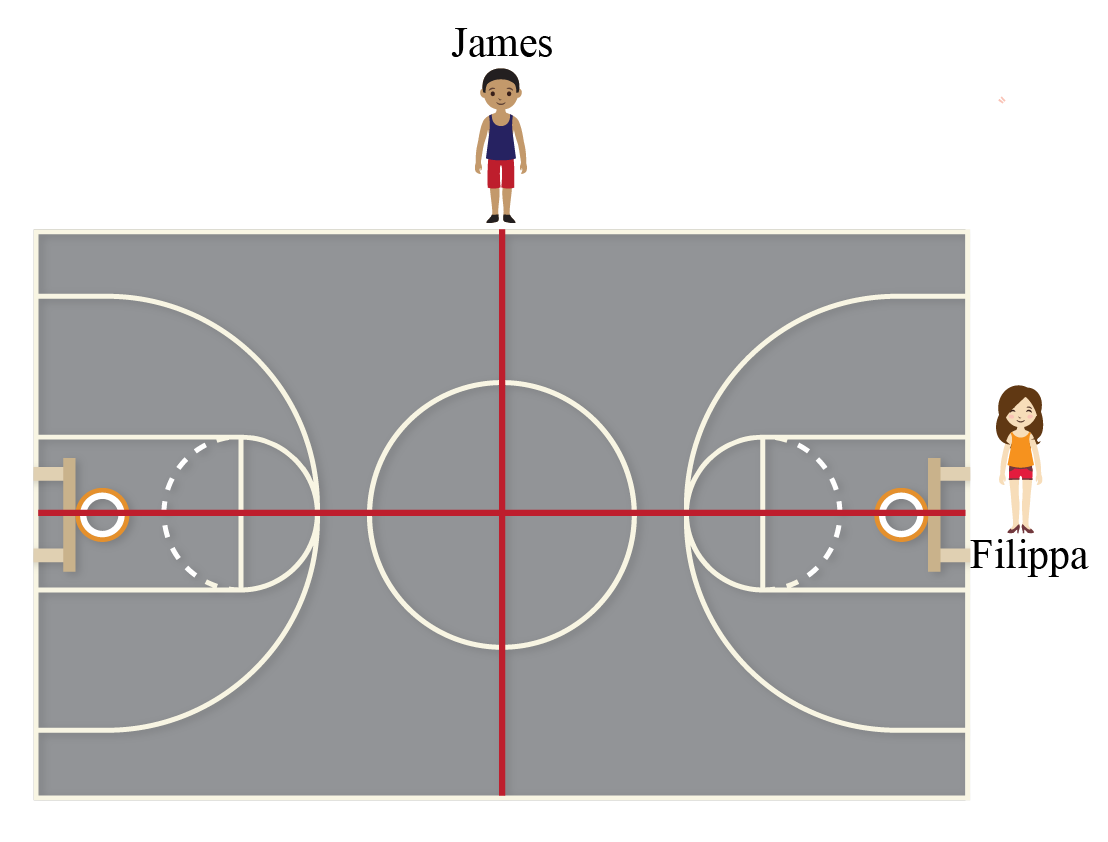James and Filippa meet after the match and James asked Filippa that what do we call these lines.

Filippa told James that these lines are called the lines of symmetry.

Let's learn about the symmetry of a circle in this session.

## How to Find If a Given Object Is Symmetrical?

Have you ever visited the Taj Mahal in India?

It is one of the most beautiful monuments ever built.

Taj Mahal is a perfect example of symmetry.If a line is drawn from the middle as shown in the above picture, we can observe that the Taj Mahal can be divided into two symmetrical parts.

We can check whether an object is symmetrical or not if we are able to divide an object into two symmetrical parts by drawing a line.

If a line divides an object into two symmetrical parts, then the object is said to be a symmetric object.

### Line of Symmetry

The line which divides any figure or shape into two identical or symmetrical shapes is called the line of symmetry of the figure.

An object can have zero lines of symmetry or it can have infinite lines of symmetry.

Explore the simulation below to check for the lines of symmetry for different shapes and figures.Think Tank
• How many lines of symmetry does a human body can have?
• Is your mirror image symmetric to you?

## What Kind of Symmetry Does a Circle Have?

A circle is symmetrical about any of its diameter.

By symmetrical, we mean that the circle can be divided into two congruent parts by any of its diameter.

Here, the circle with center $$O$$ is symmetrical about its diameter $$AB$$.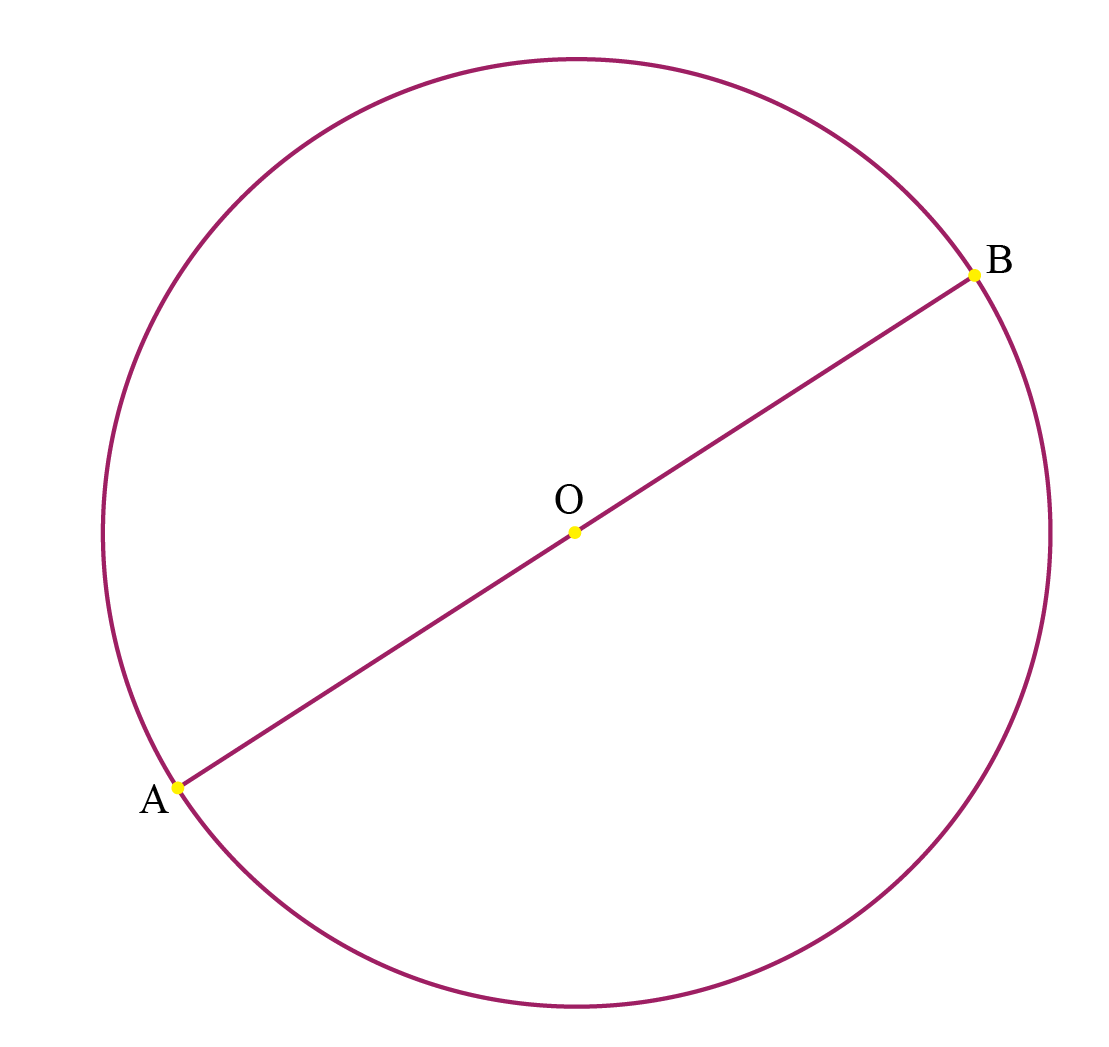A circle has rotational symmetry and the order of the symmetry in a circle is infinite.

It means that we can rotate the circle by any degree angle along its diameter.

It will always be symmetrical around the diameter.

Explore the simulation to check for the lines of symmetry in a circle.

## How Many Lines of Symmetry Does a Circle Have?

A circle has its diameter as the line of symmetry, and a circle can have an infinite number of diameters.

Hence, a circle has infinite lines of symmetry.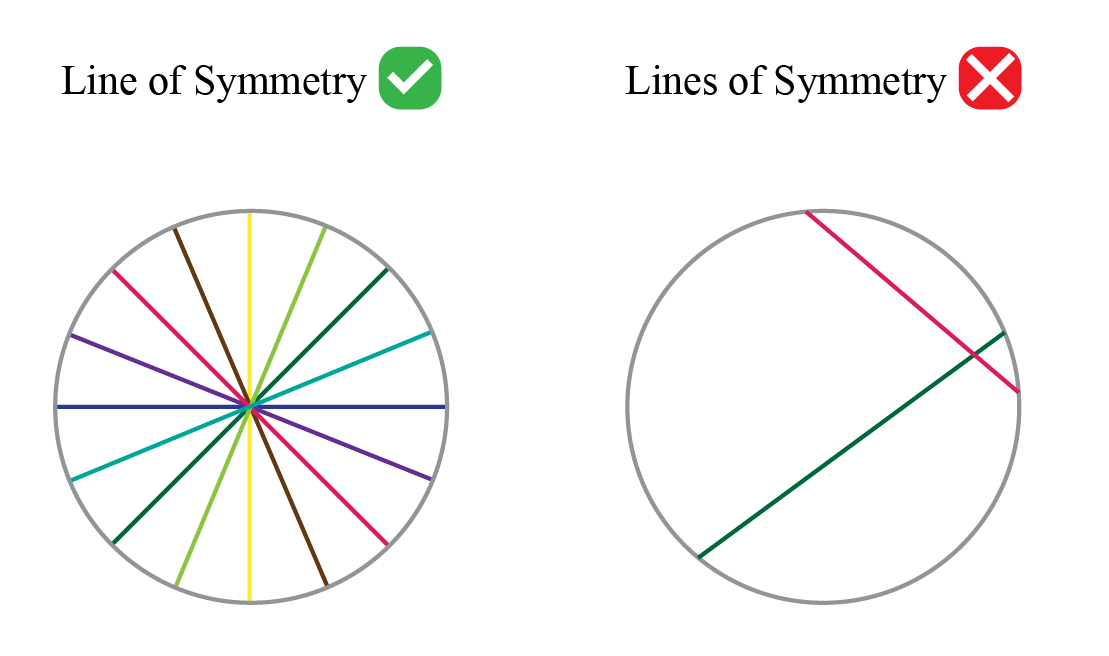Example 1

Kristine told her friends that a chord of a circle can be a line of symmetry of a circle.

Is she right?

Solution

The diameter is the line of symmetry for a circle.

The diameter is the biggest chord of the circle.

Hence a chord can be the line of symmetry of a circle only if it is a diameter.

 $$\therefore$$ Kristine is right.

## Interactive Questions on Symmetry of Any Circle

Here are a few activities for you to practice. Select/Type your answer and click the "Check Answer" button to see the result. In Q. 3, remove fullstop at the end of the ques and check the spelling of concentric.

## Let's Summarize

This mini-lesson introduced you to the fascinating concept of symmetry of any circle. The math journey around symmetry of any circle starts with what a student already knows, and goes on to creatively crafting a fresh concept in the young minds. Done in a way that is not only relatable and easy to grasp, but also will stay with them forever.

## About Cuemath

At Cuemath, our team of math experts are dedicated to making learning fun for our favorite readers, the students! Through an interactive and engaging learning-teaching-learning approach, the teachers explore all angles of a topic. Be it simulations, online classes, doubt sessions, or any other form of relation, it’s the logical thinking and smart learning approach that we at Cuemath believe in.

## 1. Can a circle be symmetrical?

Yes, a circle is symmetric about its diameter and a circle can have infinite lines of symmetry.

## 2. Which shape has only one line of symmetry?

An isosceles triangle has one line of symmetry.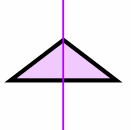No regular polygon shape has only one line of symmetry.

They always have more than one line of symmetry.

## 3. Where can you find symmetry in nature?

Symmetry can be found in many places around us.

For example, a butterfly is a symmetric creature and the Taj Mahal is a perfect example of symmetry.

## 4. Is the circle symmetric to the origin?

Yes, a circle is symmetric to the origin if we draw the axis of symmetry passing through the origin.

Then, the circle is symmetric about that axis.

Download Circles Worksheets
Circles
grade 9 | Questions Set 2
Circles
grade 9 | Questions Set 1
Circles
grade 9 | Answers Set 1
Circles
grade 9 | Answers Set 2

More Important Topics
Numbers
Algebra
Geometry
Measurement
Money
Data
Trigonometry
Calculus